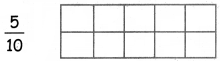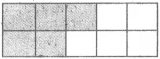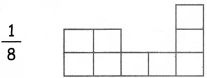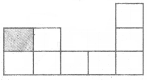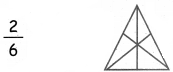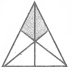Tamilnadu State Board New Syllabus Samacheer Kalvi 4th Maths Guide Pdf Term 2 Chapter 6 Fraction Ex 6.5 Textbook Questions and Answers, Notes.

## Tamilnadu Samacheer Kalvi 4th Maths Solutions Term 2 Chapter 6 Fraction Ex 6.5

I. Write the fraction for the shaded portions of the given figures.

(i)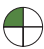$$\frac { 1 }{ 4 }$$(ii)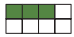$$\frac { 3 }{ 8 }$$

(iii)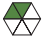$$\frac { 2 }{ 6 }$$(iv)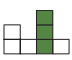$$\frac { 3 }{ 7 }$$

(v)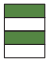$$\frac { 2 }{ 4 }$$II. Shade the figure according to the fraction

Question 1.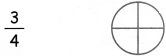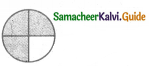Question 2.